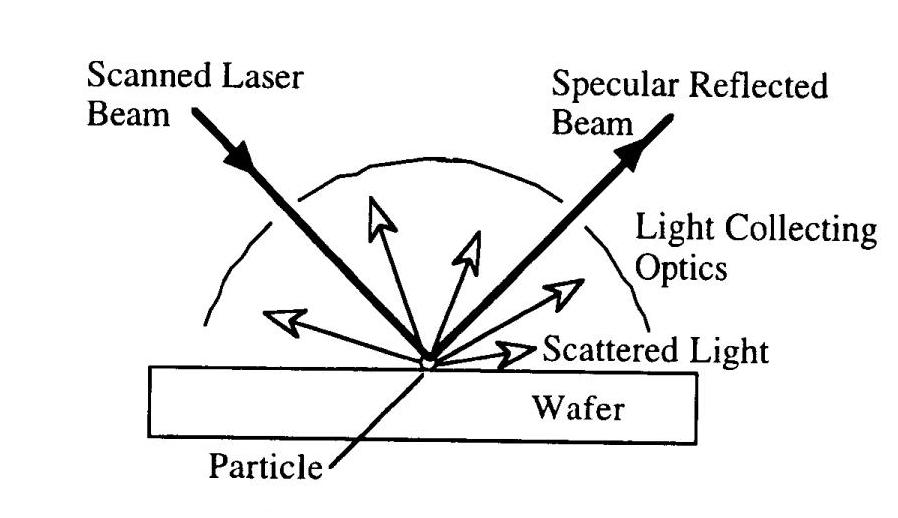# Light Scattering

## Light Scattering

The instrument that use light scattering technique is scatteromety. It is the elastic scattering of light from particles and from surface with random or periodic variations from a perfectly smooth plane. The commonly used in semiconductor characterization is to detect particles on surface. The schematic measurement arrangement is shown in Fig.1.Fig. 1 Light Scattering experimental schematic

When the focus laser beam is scaned across the sample surface, the scattered light is detected. The particles in surface scatter light in all direction. Therefore optical detector are placed at various lacations around the system to capture light as much as possible. For a particles in isolation, the scattered light is proportional to the optical scattering cross section given by$\sigma = \frac{\pi ^{4}}{18}\frac{D^{6}}{\lambda^{4}}(\frac{K-1}{K+2})^{2}$  ......................(1)

When D is the particle diameter,$\lambda$ is the laser wavelength and K is the relative dielectric constant of the particle. It is valid for D <<$\lambda$ .The particle size is determined through the size dependence of scattered light as given by Eq.(1). Smaller particles scatterless light than bigger particles. Parricles can be smaller than the wavelength of the light and still be detected. However, their size can’t be determined without recourse to calibrated standards, which are usually calibrated latex or Si sphere.

[Ref.] D.K. Schroder,Semiconductor material and device characterization, 2ndedition,John Wiley & Son,1998.